# How to calculate compound interest in Excel: daily, monthly, yearly compounding

The tutorial explains the compound interest formula for Excel and provides examples of how to calculate the future value of the investment at annual, monthly or daily compounding interest rate. You will also find the detailed steps to create your own Excel compound interest calculator.

Compound interest is one of the basic building blocks in banking and one of the most powerful financial forces around that determine the outcome of your investments.

Unless you are an accounting graduate, financial analyst or an experienced investor, it might be a bit difficult to grasp the concept from specialized financial books and manuals. The aim of this article is to make it easy : ) You will also learn how to use a compound interest formula in Excel and create a universal compound interest calculator for your own worksheets.

## What is compound interest?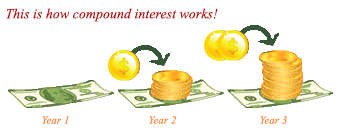In very simple terms, compound interest is the interest earned on interest. More precisely, compound interest is earned on both the initial deposit (principal) and the interest accumulated from previous periods.

Perhaps, it might be easier to start with simple interest that is calculated only on the principal amount. For example, you put \$10 into a bank account. How much will your deposit be worth after one year at an annual interest rate of 7%? The answer is \$10.70 (10 + 10*0.07 = 10.70), and your earned interest is \$0.70.

In case of compound interest, the principal in each time period is different. The bank won't give the earned interest back to you, instead they add it to your principal investment. This increased amount becomes the principal for the next time period (compounding period) and also earns interest. In other words, you earn interest not only on the principal amount, but also on the interest earned in each compounding period.

In our example, in addition to the principal amount of \$10, the earned interest of \$0.70 will also earn interest next year. So, how much will your \$10 deposit be worth after 2 years at the annual interest rate of 7% compounded yearly? The answer is \$11.45 (10.7 + 10.7*0.07 = 11.45) and your earned interest is \$1.45. As you see, at the end of the second year, not only did you earn \$0.70 on the initial \$10 deposit, you also earned \$0.05 on the \$0.70 interest that accumulated in the first year.

There are several ways to calculate compound interest in Excel, and we are going to discuss each one in detail.

## How to calculate compound interest in Excel

Long time investments can be an effective strategy to increase your wealth, and even small deposits can make a big difference over time. The Excel compound interest formulas explained further will help you get the savings strategy to work. Eventually, we are going to make a universal formula that calculates the future value with different compounding periods - daily, weekly, monthly, quarterly, or yearly.

### Calculating annual compound interest in Excel

To understand the idea of compound interest better, let's begin with a very simple example discussed at the beginning of this tutorial and write a formula to calculate annual compound interest in Excel. As you remember, you are investing \$10 at the annual interest rate of 7% and want to know how yearly compounding increases your savings.

#### Annual compound interest - formula 1

An easy and straightforward way to calculate the amount earned with an annual compound interest is using the formula to increase a number by percentage:

`=Amount * (1 + %)`.

In our example, the formula is:

`=A2*(1+\$B2)`

Where A2 is your initial deposit and B2 is the annual interest rate. Please pay attention that we fix the reference to column B by using the \$ sign.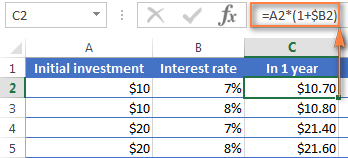As you remember, 1% is one part of a hundred, i.e. 0.01, so 7% is 0.07, and this is how percentages are actually stored in Excel. Keeping this in mind, you can verify the result returned by the formula by performing a simple calculation of 10*(1+0.07) or 10*1.07 and make sure that your balance after 1 year will be \$10.70 indeed.

And now, let's calculate the balance after 2 years. So, how much will your \$10 deposit be worth in two years' time at an annual interest rate of 7%? The answer is \$11.45 and you can get it by copying the same formula to column D.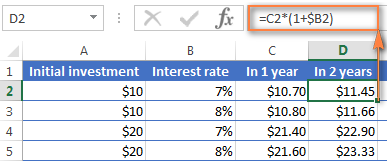To calculate how much money you will find in your bank account at the end of 3 years, simply copy the same formula to column E and you will get \$12.25.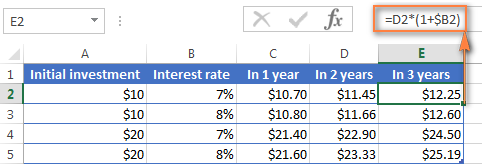Those of you who have some experience with Excel formulas have probably figured out that what the above formula actually does is multiplying the initial deposit of \$10 by 1.07 three times:

`=10*1.07*1.07*1.07=12.25043`

Round it to two decimal places and you will get the same number as you see in cell E2 in the screenshot above - \$12.25. Naturally, you can directly calculate the balance after 3 years using this formula:

`=A2*1.07*1.07*1.07`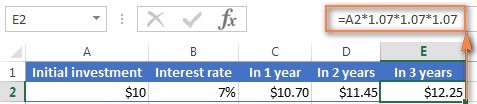#### Annual compound interest - formula 2

Another way to make an annual compound interest formula is to calculate the earned interest for each year and then add it to the initial deposit.

Assuming that your Initial deposit is in cell B1 and Annual interest rate in cell B2, the following formula works a treat:

`=B1 + B1 * \$B\$2`For the formula to work correctly, please mind the following details:

• Fix the reference to the Annual Interest Rate cell (B2 in our case) by adding the \$ sign, it should be an absolute column and absolute row, like \$B\$2.
• For Year 2 (B6) and all subsequent years, change the formula to:
Year 1 balance + Year 1 balance * Interest Rate

In this example, you'd enter the following formula in cell B6 and then copy it down to other rows, like demonstrated in the screenshot below:

`=B5 + B5 * \$B\$2`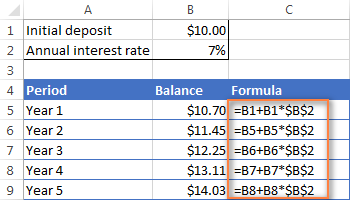To find out how much interest you actually earned with annual compounding, subtract the Initial deposit (B1) from Balance after 1 year (B5). This formula goes to C5:

`=B5-B1`

In C6, subtract Balance after 1 year from Balance after 2 years, and drag the formula down to other cells:

`=B6-B5`

You should see the earned interest growth like in the screenshot below.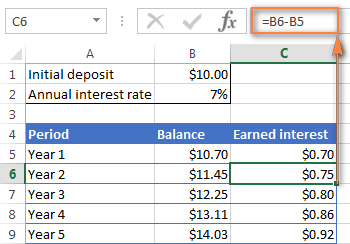The above examples do a good job illustrating the idea of compound interest, don't they? But none of the formulas is good enough to be called a universal compound interest formula for Excel. Firstly, because they do not let you specify a compounding frequency, and secondly, because you have to build an entire table rather than simply enter a certain duration and interest rate.

Well, let's take a step forward and create a universal compound interest formula for Excel that can calculate how much money you will earn with yearly, quarterly, monthly, weekly or daily compounding.

### General compound interest formula

When financial advisors analyze the impact of compound interest on an investment, they usually consider three factors that determine the future value of the investment (FV):

• PV - present value of the investment
• i - interest rate earned in each period
• n - number of periods

By knowing these components, you can use the following formula to get the future value of the investment with a certain compounded interest rate:

FV = PV * (1 + i)n

To illustrate the point better, here are a couple of quick examples.

#### Example 1: Monthly compound interest formula

Suppose, you invest \$2,000 at 8% interest rate compounded monthly and you want to know the value of your investment after 5 years.

First off, let's write down a list of components for your compound interest formula:

• PV = \$2,000
• i = 8% per year, compounded monthly (0.08/12= 006666667)
• n = 5 years x 12 months (5*12=60)

Input the above numbers in the formula, and you will get:

`= \$2,000 * (1 + 0.8/12)5x12 `

or

`= \$2,000 * 1.00666666760`

or

`= \$2,000 * 1.489845708 = \$2,979.69`

#### Example 2: Daily compound interest formula

I hope the monthly compound interest example is well understood, and now you can use the same approach for daily compounding. The initial investment, interest rate, duration and the formula are exactly the same as in the above example, only the compounding period is different:

• PV = \$2,000
• i = 8% per year, compounded daily (0.08/365 = 0.000219178)
• n = 5 years x 365 days (5*365 =1825)

Supply the above numbers into the compound interest formula, and you will get the following result:

`=\$2,000 * (1 + 0.000219178)1825 = \$2,983.52`

As you see, with daily compounding interest, the future value of the same investment is a bit higher than with monthly compounding. This is because the 8% interest rate adds interest to the principal amount each day rather than each month. As you can guess, the monthly compounding result will be higher than annual compounding.

All this is good, but what you really want is an Excel formula for compound interest, right? Just bear with me for a little longer, please. Now we are getting to the most interesting part - building your own powerful and versatile compound interest calculator in Excel.

### Compound interest formula in Excel (daily, weekly, monthly, yearly compounding)

Usually, there is more than one way to do something in Excel and a compound interest formula is not an exception :) Although Microsoft Excel provides no special function for calculating compound interest, you can use other functions to create your own compound interest calculator.

Let's start creating our Excel compound interest calculator with entering the basic factors that determine the future value of an investment in an Excel worksheet:

• initial investment (B3)
• annual interest rate (B4)
• number of compounding periods per year (B5)
• number of years (B6)

When done, your Excel sheet may look similar to this: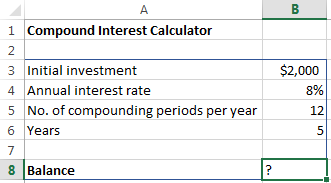All you need now is the compound interest formula to calculate the earned amount (Balance) based on the input values. The best news is that you don't have to re-invent the wheel. We will simply take the time-tested compound interest formula used by banking and other financial institutions and translate it into Excel's language.

Compound interest formula for Excel:

Initial investment * (1 + Annual interest rate / Compounding periods per year) ^ (Years * Compounding periods per year)

For the above source data, the formula takes this shape:

`=B3 * (1 + B4 /B5) ^ (B6 * B5)`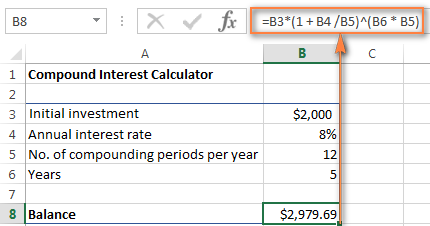The numbers look rather familiar? Yep, these are the same values and calculations that we've performed with a monthly compound interest formula, and the result proves that we did everything right!

If you want to know how much your investment will be worth at an 8% annual interest rate compounded quarterly, simply enter 4 in cell B5: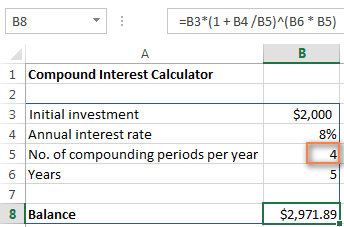To calculate the future value of your investment with semi-annual compounding, enter 2 as the Compounding periods per year value. For weekly interest rates, enter 52, this is how many weeks each year contains. If you are interested in daily compounding, enter 365, and so on.

To find the amount of earned interest, simply compute the different between the future value (balance) and the present value (initial investment). In our case, the formula in B9 is as simple as:

`=B8-B3`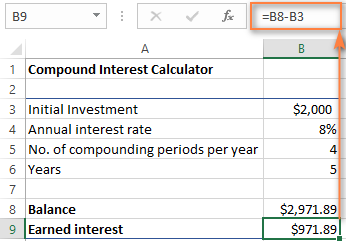As you see, we've created a truly universal compound interest calculator for Excel. Hopefully, now you have no regrets that you invested a few precious minutes in figuring out the tricky compound interest formula used by financial planners : )

### Advanced compound interest calculator for Excel

If for some reason you are not quite happy with the above approach, you can create your Excel compound interest calculator using the FV function that is available in all versions of Excel 2000 to 2019.

The FV function calculates the future value of an investment based on the input data similar to the ones we've discussed, though its syntax is a bit different:

FV(rate, nper, pmt, [pv], [type])

The detailed explanation of the arguments can be found in the Excel FV function tutorial.

In the meantime, let's build a FV formula using the same source data as in monthly compound interest example and see whether we get the same result.

As you may remember, we deposited \$2,000 for 5 years into a savings account at 8% annual interest rate compounded monthly, with no additional payments. So, our compound interest formula goes as follows:

`=FV(0.08/12, 5*12, ,-2000)`

If you need some explanation of the parameters, here you go:

• rate is 0.008/12 since you have the 8% annual interest rate compounded monthly.
• nper is 5*12, i.e. 5 years * 12 months
• pmt is left blank because we have no additional payments.
• pv is -2000 since it's an outflow and should be represented by a negative number.

Enter the above formula in an empty cell, and it will output \$2,979.69 as the result (which is perfectly inline with the result of the math calculation performed in the monthly compound interest example).

Naturally, nothing prevents you from replacing the values with cell references:

`=FV(B4/B5, B6*B5, , -B3)`

The screenshot below shows the future value of \$4,000 investment after 15 years at an annual interest rate of 7% compounded weekly: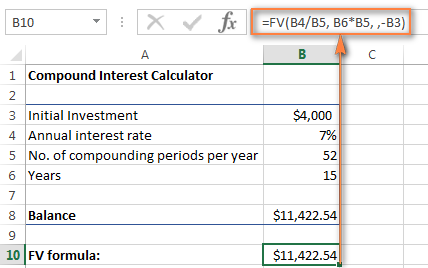To make your Excel compound interest calculator even more powerful, you can extend it with the Additional contributions option (additional payments) and modify the compound interest formula accordingly.

`=FV(B4/B5, B6*B5, -B8, -B3, B9)`

Where:

• B3 - principal investment
• B4 - annual interest rate
• B5 - the number of compounding periods per year
• B6 - the number of years to save
• B8 - additional contributions (optional)
• B9 - additional contributions type. Remember that you enter 1 if you deposit an additional amount at the beginning of the compounding period, 0 or omitted if additional payments are made at the end of the period.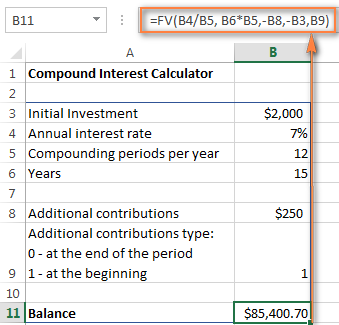If you are curious to try this advanced compound interest calculator for Excel to compute your savings, you can download it at the end of this post.

Tip. Another quick way to calculate compound interest is by doing What-If analysis with the help of Excel data table.

## Compound interest calculators online

If you prefer investing money rather than time in figuring out how to calculate compound interest in Excel, online compound interest calculators may come in handy. You can find plenty of them by entering something like "compound interest calculator" in your preferred search engine. In the meantime, let me quickly present a couple of my favorite ones.

#### Compound interest calculator by Bankrate

The key benefits of Bankrate compound interest calculator is ease-of-use and visual presentation of the results. This calculator lets you enter the savings inputs manually in boxes or by moving a slider. As you do this, the estimated total is displayed on top and immediately reflected in the graph below: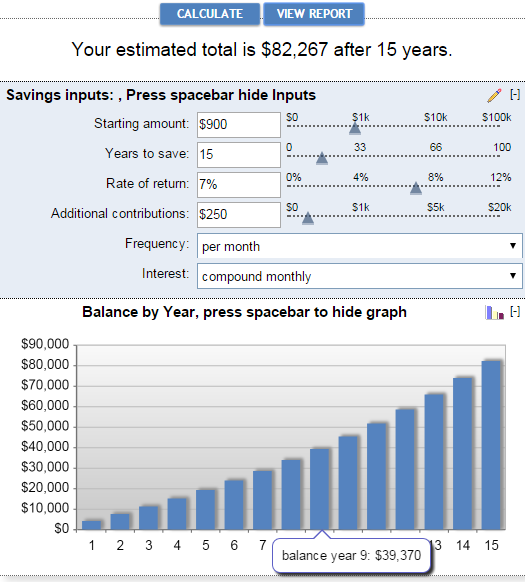Clicking the View Report button generates a "Summary Report" as well as "Savings Balance" that provides the detailed info on the amount of additional contributions, earned interest and balance for each year.

#### Compound interest calculator by Money-Zine

The online calculator from Money-Zine is much simpler compared to Bankrate's one. It asks you to specify only 3 values: the principal investment, interest rate and duration. As soon as you supply these numbers and click the Calculate button, it will show you all types of compound interest rate (daily, weekly, monthly, annual, etc.) as well as the future values with a corresponding compounding.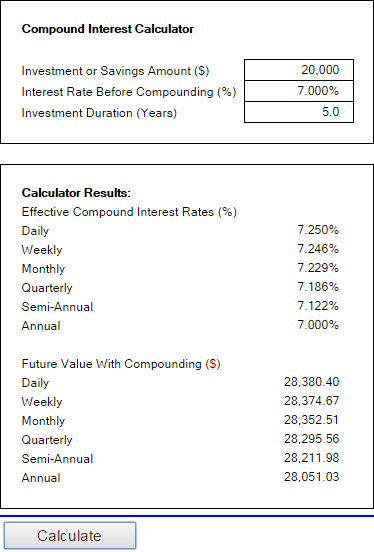#### Compound interest calculator by MoneySmart

This is a really nice online compound interest calculator run by Australian Securities and Investments Commission. It lets you input all relevant factors that determine the future value of your investment and outputs the result as a graph. By hovering over a certain bar in the graph, you can see the summary info for that particular year.This is how you calculate compound interest in Excel and outside it :) I hope at least one compound interest formula discussed in this article has proved helpful to you. Anyway, I thank you for reading and hope to see you on our blog next week!

Compound interest calculator for Excel (.xlsx file)

## You may also be interested in

1. sir please send me DDA calculator daily deposit scheme interest calculator suppose any per giveing 100/ rs daily for 180 days @ 7% per annum for 180 days. how we calculate daily interest and total amount i have to pay

thanks with regard

2. sir please send me DDA calculator daily deposit scheme interest calculator suppose any per giveing 50/ rs daily for 90 days @ 7% per annum for 90 days. how we calculate daily interest and total amount i have to pay

thanks with regard

3. Brilliant. Thanks a lot, exactly what I needed.

4. I have 2 situations.

1) I have 200,000 and it will be double in 10 years time. What compound interest rate would be required? Solution in excel please.

2) PV of cash out flow for 48 months @ 1500 per month paid at the beginning of each month. Solution in excel please.

5. What if I need to find the future value when it has a monthly investment? EX: Inital Investment \$8000 , Monthly Investment \$125, Rate 8.75, and 20 years.

6. How do I begin to solve this problem or set up a formula in exel

5.Suppose John could change investment plans at E-Trade and earn an additional 1% per annum beginning in year 2 and an additional 1% beginning in year 4. How much money do they need to save each month to reach their goal?

7. it is very help full formula please send more formula

8. Suppose you invest Rs.10000 in a business each December 31 (at the end of each year) for the next 10 years. If you are getting interest of 12%, compounded semiannually, how much will you have after 10 years?

Question Hint
invest Rs.10000 in a business each December 31 (at the end of each year) for the next 10 years?

9. Hi Svetlana, can you help with a formula (for Excel) to "reverse engineer" compound interest. I have an investment that compounds weekly, but at no set rate (trading currencies, some weeks are better than others). I want to work out each week, what the effective compound interest rate has been since starting the investment, and then use that rate to work out a "what if" scenario..... what if this investment continues to perform at the current level till the end of the financial year?

So, I want to enter the Initial Value, Current Value, Number of Periods since start, and it should give me the "effective weekly compound interest rate".

Thank you.

10. Hi,

I found this site very helpful until I found an error in the way you work with the FV function.

I cross referenced the ASIC calculator with your formula and your excell formula was only working when the number of compounding periods equals exactly 12. As soon as you change this figure it becomes very inaccurate.

This is because in your formula the contributions are only made the same number of times that interest is compounded. Ie. when you set pmt to \$250 and compounding periods as 12, then it adds the \$250 12 times, when you set pmt to \$250 and number of compounding periods to 1 then it is mistakenly only adding the \$250 once instead of 12 times.

You can fix this by modifying your formula;
=FV(B4/B5, B6*B5,-B8,-B3,B9)

=FV(B4/B5, B6*B5,-B8*12/B5,-B3,B9)

11. sir plz send daily deposit scheme (dds) formula

1) Joint Venture

\$24,000 investment
\$10,000 gain in 45 days
Gaining the same interest every 45 days effective February 18, 2015 to current date.

2) 1) Joint Venture

\$24,000 investment
\$10,000 gain in 45 days
Gaining the same interest every 90 days effective February 18, 2015 to current date.

13. Excel is one of those tool-case programs that is indeed useful for everyone - in the same way that 1-2-3 originally was. It's not that Office (as a whole) isn't useful - it is; it's just that it gets taken for granted. How many of you use Office (not just Word or even Excel) for non-work-related things at home on a weekly basis?

14. dears,

if the initial investment is 1,000,000 for ten years with 200% accumulated interest ( 2,000,000 )

how can calculate the compound interest rate ???

for this case the compound interest is 11.6123174 %

thanks

15. Hi,

Thank you for this post. Can you please tell me the formula in excel to calculate n (years) if the amount, rate and principal is known.

16. Do large financial institutions/banks use 365.25 for annual rates (do to leap years)?

17. Never saw an answer to Richards question (Ref. #9) Please respond to it, email me the answer. I am also trying to see the answer to help my Son!

18. I'm trying to find the annual return for a string of 12 monthly returns, any chance someone knows what the formula would be?

For example:

-2.75% -1.92% 1.91% 1.13% 0.96% 1.82% 1.22% 0.85% 0.39% -1.27% 2.81% 1.08%

Jan E5 -2.75%
Feb F5 -1.92%
Mar G5 1.91%
Apr H5 1.13%
May I5 0.96%
Jun J5 1.82%
Jul K5 1.22%
Aug L5 0.85%
Sep M5 0.39%
Oct N5 -1.27%
Nov O5 2.81%
Dec P5 1.08%

19. I am trying to work out what the monthly payments would be on a loan with compound interest.

i.e. PV 1,000,000 - annual rate 5% - for 12 months

I am able work out the compound value at the end of the term using FVSCHEDULE, but how do I work out what how the monthly payments will impact this?

Thank you

20. Hi
I am trying to calculate the amount owing on a loan of \$6,600 taken out 1/01/1990 which went for 2.5 years (no interest was paid), and then \$4,150 was paid back but the remaining \$2,450 still outstanding. Interest calculated daily (as per bank loan).
I have looked up the bank interest rates for the period so can use a pivot table but would like the formula to calculate what is still owed.

thanks

21. Investment analysis:
Initial Investment: 300,000,000
Cost of Capital: 10%
Assume: (yearly gross revenue): 32,964,000 will not increase over the 20-year period.
Assume: 360,000 expense for 1st year and will increase with annually compounded rise of expense at 7.25%
Questions: 1)compute the payout(payback) point for undiscounted cash. 2) compute the payout(payback) point for discounted (time value) cash.

22. My question is
loan 500000
Loan percent 10%
time 60 month
Interest calculation-daily

After 365 days the accrued interest should automatically capitalized.(if interest not paid)
it means if interest not paid, the accrued interest of 48767 must capitalized
Now the total principal is 548767. the interest must calculate on this figure.
and if repay the the loan first settled capitalized interest, then remaining interest and then principal.

Now it's 400 days running.
our statement shows:
Total loan - 548767
Accrued interest (after 365 Days again)- say 21000

If The loan amount partially with 100000.

First settle capitalized interest i.e 48767
then settled accrued interest i.e. 21000, then remaining amount to be deducted on Principal.
after that principal amount will be 469676 and interest will be calculated on it.

23. Hi,

I just wanted to say a BIG Thank You for creating this "learn-and-do-it-yourself" website.

Luv yu all

24. can u explain how to find out rate of compounded
interest? Example. The interest earned by a principal invested 5% compound anually for 3years is computed using the relation c=Prt, where c is the interest, P is the principal, r is the rate, and t is the time.

25. Can anyone tell me how to calculate the interest that i am paying if: I have an amount of principal that i am paying interest on and the amount increases every period and i know what the total amount of interest i am paying at the end is?

26. Hi

I want to build a tool to calculate required monthly instalments, when interest rate is fixed, future value is known/changeable, duration is known/ changeable and interest is compound daily.

Anyone any ideas?

27. The following formula expresses interest compounded at 4.5% quarterly on a debt of £1,000.00:
SUM=(£1,000*(1+0.045)^(U9*4))
where U is the fraction of the year the invoices was paid late (days late/365)

How do I amend the formula such that interest is calculated not with reference to the quarters BUT with reference to the annual quarter days found in many leases namely: 25 March, 24 June, 29 September and 25 December annually?

28. I have a client that experienced embezzlement from a former employee. How can I calculate interest on the money he embezzled? Can I continue to add new amounts as they happen and continue to calculate interest on the entire amount.

Time period of theft 2011-2014. Money has not been recovered so I want to continue to calculate interest.

Thank you

29. re above query
\$1,000 loaned November 9, 2015
interest is 5.2%
calculated Monthly
end date of loan is March 10, 2016
no payment have been made on this loan

30. Maria, great website. Thank you
I need to calculate the accumulated interest on a loan between two dates. Interest rate is 5.2% calculated monthly but there have been no payments.

Amount Start End Int Calculated Total
\$1,000 9-Nov-15 10-Mar-16 5.20% Monthly ?
\$5,000 26-Nov-15 10-Mar-16 5.20% Monthly ?
\$6,700 1-Dec-15 10-Mar-16 5.20% Monthly ?

31. Hi,

In my case, I would like to know the compound interest.

For example, every month I am paying a particular amount for 20 months.
I have the future value. So is there a way to know the compound the interest?

Future value = 2,250
period = 20 months
monthly amount = 75

What will be the Compound interest?

Thanks,
Anand

32. I was wondering if anyone could help me with this problem. I'm trying to calculate the future value of a 1 year investment using excel but I am having issues because I have two differing semiannual interest rates.
Here's what I have:
Time period= 1 year
Principal=\$15000
1st Semiannual rate=4.80%
2nd Semiannual rate=6.93%
monthly payments =\$100

33. I have 135 after 3 years on an initial capital of 100
What would be my compound interest per anum

34. Hi Maria,

I want to know the formula for the following calculation.

Monthly deposit = 1000
Monthly interest= 1%
Compounding frequency = once a year till 5 years

Ex: first month interest = 1000*1% , second month interest = (1000+1000)*1% so total interest for that year = 780. so need to calculate simple interest till 12 months and total principal+simple interest will become principal for the next year(need to compound annually)

35. Hi,
I am hoping that you can assist with the following:-

I have a Loan with the following parameters:-
Principal: \$200,000, Annual Interest Rate: 5%, Loan Period: 25 years, Payments being made monthly. Using Excel's PPMT formula I calculated a Monthly Repayment of \$1,169.18 =-PMT(5/12,25*12,200000).

What I want to do now is to calculate the number of repayments if I increase the calculated monthly Repayment by a constant amount, say \$50.00 per month.

I tried using Excel's NPER and I tested it using the same inputs from above. NPER(rate,pmt,pv,[fv],[type])or NPER(5/12,1169.18,200000,0). This gave me a result of 129.41 whereas I was expecting 25years X 12 = 300months. So unless I have erred then NPER is not what I want. At this point I started trying to transpose the Compound Interest formula with little luck.

So my question is:-
"How do I calculate the Term of a loan when the Monthly Repayment Value, The Annual Interest Rate and the Original Loan Amount are all known?"

• Hello, Danny,

Looks like minus is missing in your formula. Please make sure you use this one:
=NPER(5%/12;-1169,18;200000)

36. sir please send me DDA calculator daily deposit scheme interest calculator suppose any per giveing 50/ rs daily for 90 days @ 7% per annum for 90 days. how we calculate daily interest and total amount i have to pay

thanks with regard

37. Hi! I do have a question, I don't know if this is complicated or not. How can I create a table that responds to the number of years as an input for the compound interest?
Example: What is the New Principal Amount in 4 years where there should be 4 rows that would show what the new principal is going to be in that particular year.

• Hello, Louie,

For us to be able to assist you better, please send us a small sample table with your data in excel to suppot@ablebits.com.

38. i could use some help.I am drowning in a on line fin 301 class. what is the formula on an excel spreadsheet for the following:
compute the present value of an \$1,850 payment made in 8 years when the discount rate is 10%.

39. Hello, is there an excel function that will be able to predict values for 25 years with an escalation rate of X% and frequency of every n years.

For example: Lease rate at the moment is \$200 with an escalation of 3% every 3 years.

Is is possible to have a formula that will auto-compute annual values?

Thank you.

• Hello, Arrey,

Please clarify what you mean by escalation of 3% every 3 years.

40. Good day.
Let's say i want to save 1000 000,i want it in 5 years time and the interest rate is 11%. What formula can i use to work out the monthly amount to save in order to get to 1000 000 in 5 years.

• Hello, Tanya,

Your initial deposit can be found using this formula:
=-PV(0,11;5;0;1000000)

Here is one to perform a reverse check:
=FVSCHEDULE(593451,33;{0,11;0,11;0,11;0,11;0,11})

41. A doubt , suppose I bought a land 5 year ago at price 25000/- and sold this year at 35000/-, then what compound interest I am getting per year,when interest is compouding every day

42. In line with Kailua's request above, I am seeking an excel formula for compounding interest with a STARTING monthly deposit of \$100 invested at 7% per year (compounded annually), and increasing the monthly deposit by 3% per year (i.e. Year 2 would see a monthly deposit of \$103.00; Year 3 would see a monthly deposit of \$106.09; Year 4 would see a deposit of \$109.27, etc.). Also, the variable of compounding intervals for daily, weekly, monthly, quarterly would be nice)

I have been unable to find such a calculator on the Internet, as all the formulas do not allow for annual donation changes by donation percentage, as I have stated above. I suspect that Kailua was seeking a similar formula.

In summary, I am planning to see what the final savings amount will be if I advise my grandchild to contribute \$100 per month to his investment portfolio (i.e. \$1200 in Year 1), and assume a 7% annual return; and increase his monthly contribution by 3% each successive year (i.e. \$103 per month in Year 2) until he retires at age 65.

Thank you............Richard

43. how we calculate compund intrest for momthly.show me all the monthly calculation for this.
term:18months
min.deposit:200
rate of int.11.5%p.a

44. Hello

You clearly love excel.
Can you help me devise a formulae to reduced monthly interest charged against a loan as additional funds were added to Initial loan. It is complication of using some housing funds as a general account.

45. 80000 amount per 7% interest how can find out

• BC Guju tu rahnay de mc

• TMC tu bhi guju ke udhar he job kar ta hai and uski he roti khata hai. yad rakh guju nahi tere khane tak ke wandhe ho jaye ge MC.

46. question. trying to calculate interest for 1 year assuming 27 periods where the first period earns the full rate of interest 10%, each period decreases the amount of interest by 2/27 the last period earns 1/27 of the full amount of interest. Trying to get an excel formula. I would appreciate ant help as soon as possible,.

47. I'm looking for an excel spreadsheet that calculates the following:

Initial Investment
Interest Rate
Monthly Deposit
Increase Deposit Annually by (Percentage)
Time Period
Future Value

Can this be done? Can there be a schedule too? I have been searching the internet high and low and cannot find anyone who has this.

• @ Kailua
if you multiply the monthly contribution type by 12 that will give you additional monthly. (ie. from example above =FV(B4/B5, B6*B5, (-B8*12), -B3, B9).

Not sure what you mean by 'Increase Deposit Annually by (Percentage) but if this is considered a rollover where 0 dividends are paid to you, the next year's starting principal will be the previous years Future value.

You would have create your own schedule and every year after the FV has been determined consider this your next years principal balance.

48. Nice Svetlana i appriciate your work i have no words for you but why not i want to say i adore you due to your work and your positive feeling & thinking

• Thank you so much for your kind words, Naveed!

• I love you

49. can u explain how to find out rate of compounded interest ?....
example.....principal
amount \$5000 invested for 1 year. Interest compounded quarterly.
Interest amount \$449.40. Principal amount + Interest amount is \$5449.40.